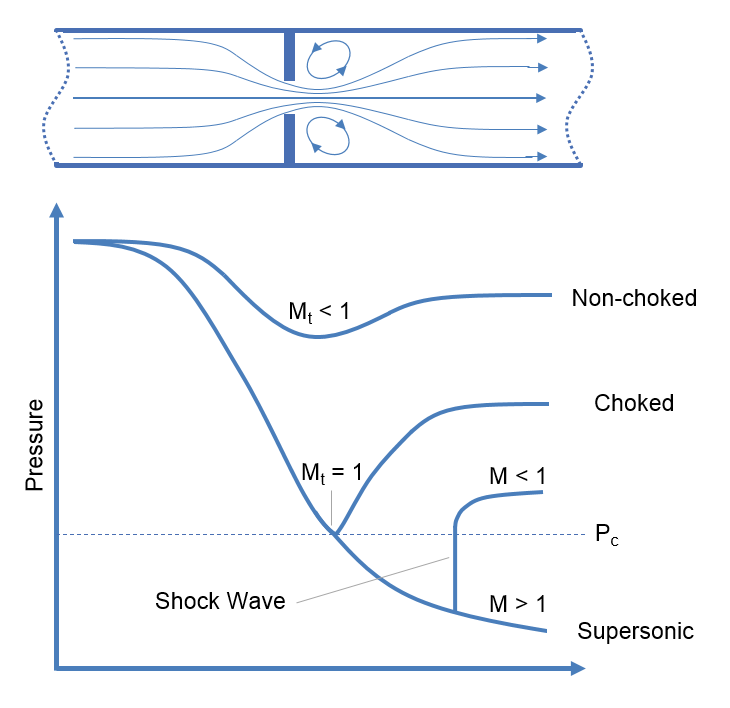f
Neutrium

# Speed of Sound

Choked FlowChoked flow is a phenomenon that limits the mass flow rate of a compressible fluid flowing through nozzles, orifices and sudden expansions. Generally speaking it is the mass flux after which a further reduction in downstream pressure will not result in an increase in mass flow rate.

Mach Number

The Mach number is the ratio of the relative velocity of a fluid to the local speed of sound. This article provides the equation for the calculation of the Mach number and a discussion of its uses.

Speed of Sound in Fluids and Fluid in Pipes

This article provides the formulae for the calculation of speed of sound in fluids and fluid filled circular pipes. The speed of sounds is important in piping systems for the calculation of choked flow for gases and pressure transient analysis of liquid filled systems.